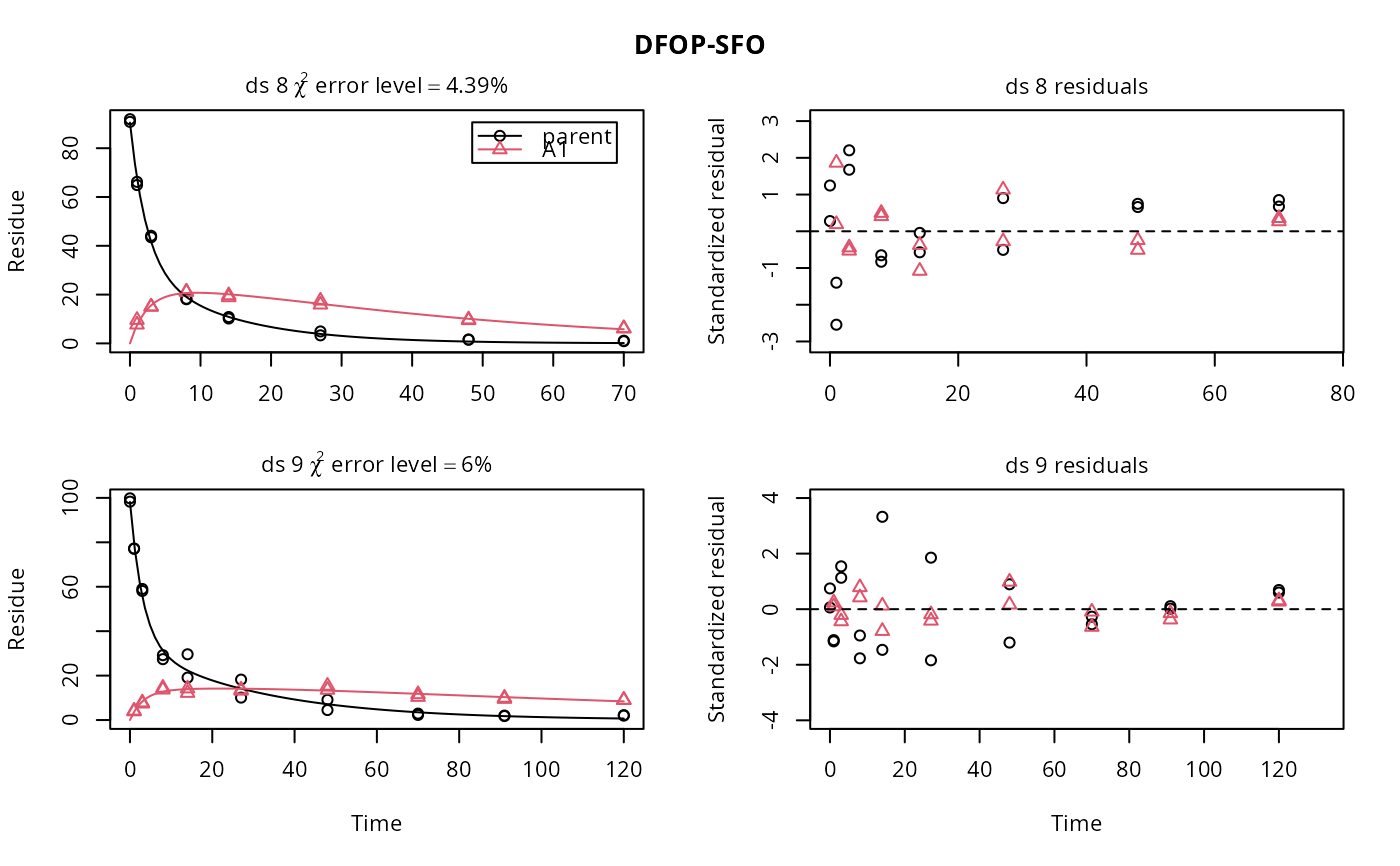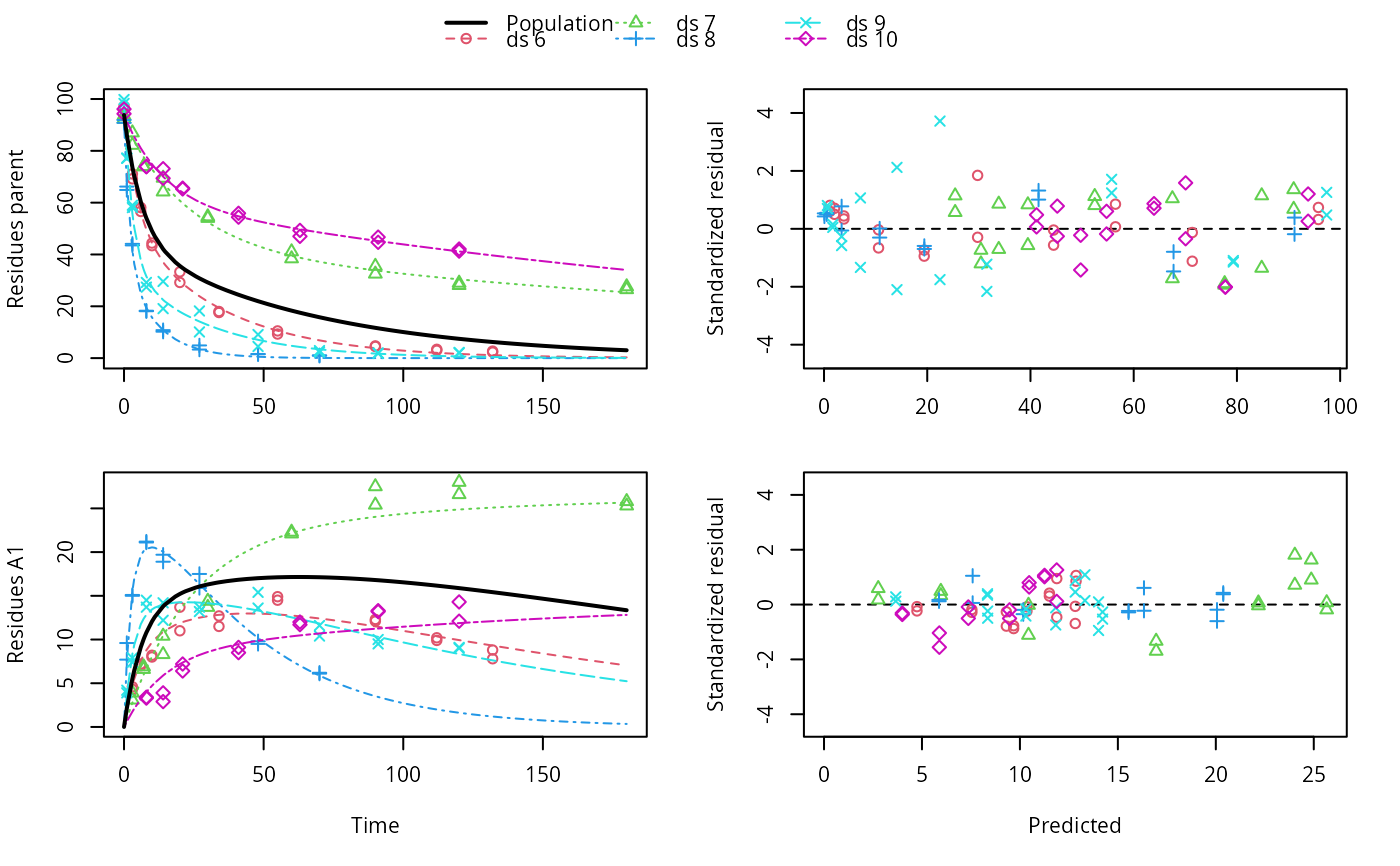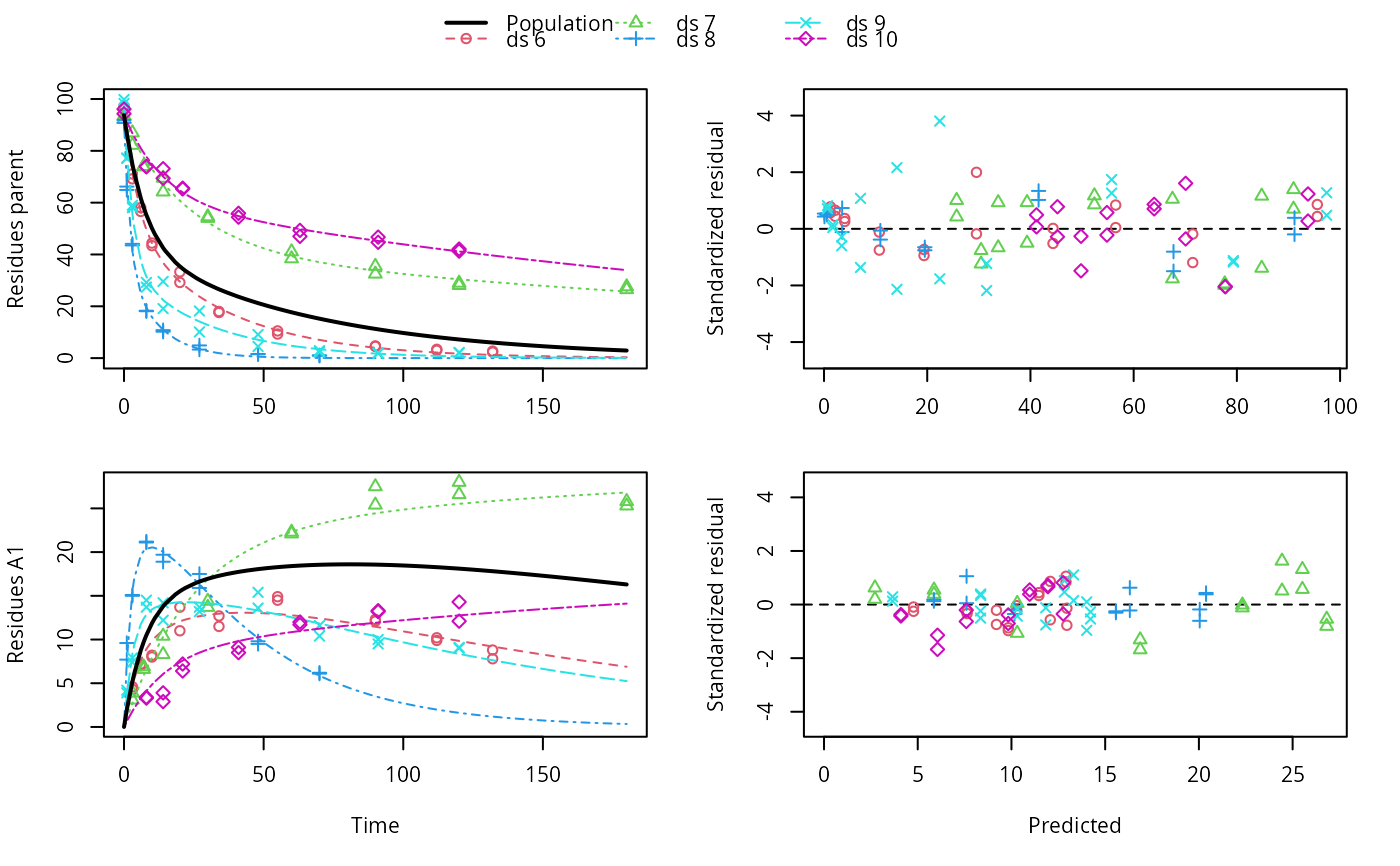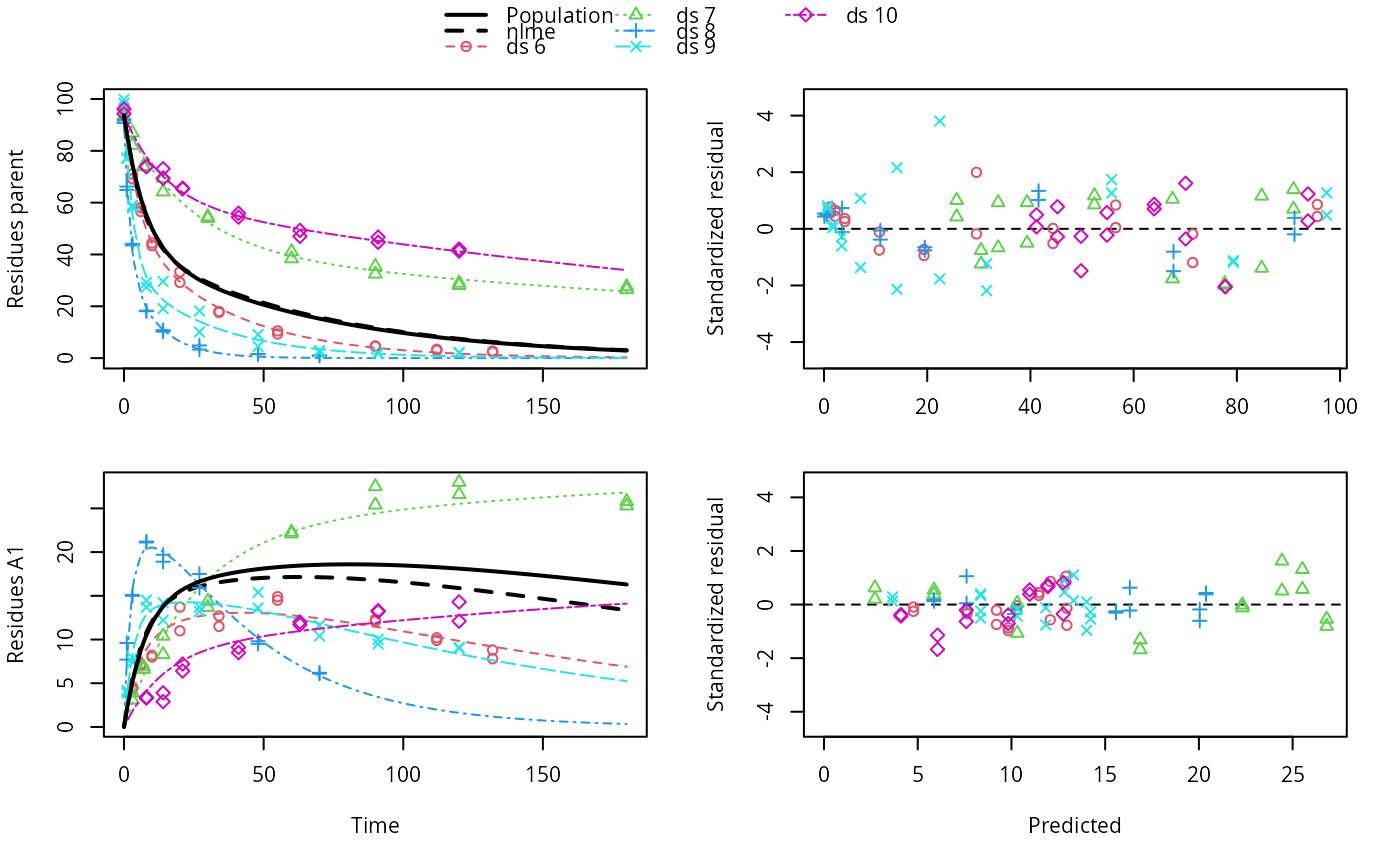Plot predictions from a fitted nonlinear mixed model obtained via an mmkin row object

# S3 method for mixed.mmkin
plot(
x,
i = 1:ncol(x$mmkin), obs_vars = names(x$mkinmod$map), standardized = TRUE, xlab = "Time", xlim = range(x$data$time), resplot = c("predicted", "time"), pred_over = NULL, test_log_parms = FALSE, conf.level = 0.6, default_log_parms = NA, ymax = "auto", maxabs = "auto", ncol.legend = ifelse(length(i) <= 3, length(i) + 1, ifelse(length(i) <= 8, 3, 4)), nrow.legend = ceiling((length(i) + 1)/ncol.legend), rel.height.legend = 0.02 + 0.07 * nrow.legend, rel.height.bottom = 1.1, pch_ds = 1:length(i), col_ds = pch_ds + 1, lty_ds = col_ds, frame = TRUE, ... ) ## Arguments x An object of class mixed.mmkin, saem.mmkin or nlme.mmkin i A numeric index to select datasets for which to plot the individual predictions, in case plots get too large obs_vars A character vector of names of the observed variables for which the data and the model should be plotted. Defauls to all observed variables in the model. standardized Should the residuals be standardized? Only takes effect if resplot = "time". xlab Label for the x axis. xlim Plot range in x direction. resplot Should the residuals plotted against time or against predicted values? pred_over Named list of alternative predictions as obtained from mkinpredict with a compatible mkinmod. test_log_parms Passed to mean_degparms in the case of an mixed.mmkin object conf.level Passed to mean_degparms in the case of an mixed.mmkin object default_log_parms Passed to mean_degparms in the case of an mixed.mmkin object ymax Vector of maximum y axis values maxabs Maximum absolute value of the residuals. This is used for the scaling of the y axis and defaults to "auto". ncol.legend Number of columns to use in the legend nrow.legend Number of rows to use in the legend rel.height.legend The relative height of the legend shown on top rel.height.bottom The relative height of the bottom plot row pch_ds Symbols to be used for plotting the data. col_ds Colors used for plotting the observed data and the corresponding model prediction lines for the different datasets. lty_ds Line types to be used for the model predictions. frame Should a frame be drawn around the plots? ... Further arguments passed to plot. ## Value The function is called for its side effect. ## Author Johannes Ranke ## Examples ds <- lapply(experimental_data_for_UBA_2019[6:10], function(x) x$data[c("name", "time", "value")])
names(ds) <- paste0("ds ", 6:10)
dfop_sfo <- mkinmod(parent = mkinsub("DFOP", "A1"),
A1 = mkinsub("SFO"), quiet = TRUE)
# \dontrun{
f <- mmkin(list("DFOP-SFO" = dfop_sfo), ds, quiet = TRUE)
plot(f[, 3:4], standardized = TRUE)# For this fit we need to increase pnlsMaxiter, and we increase the
# tolerance in order to speed up the fit for this example evaluation
# It still takes 20 seconds to run
f_nlme <- nlme(f, control = list(pnlsMaxIter = 120, tolerance = 1e-3))
plot(f_nlme)f_saem <- saem(f, transformations = "saemix")
plot(f_saem)f_obs <- mmkin(list("DFOP-SFO" = dfop_sfo), ds, quiet = TRUE, error_model = "obs")
f_nlmix <- nlmix(f_obs)
#> Error in nlmix(f_obs): could not find function "nlmix"
plot(f_nlmix)
#> Error in plot(f_nlmix): object 'f_nlmix' not found

# We can overlay the two variants if we generate predictions
pred_nlme <- mkinpredict(dfop_sfo,
f_nlme$bparms.optim[-1], c(parent = f_nlme$bparms.optim[], A1 = 0),
seq(0, 180, by = 0.2))
plot(f_saem, pred_over = list(nlme = pred_nlme))# }